# Quiz 10: The Political Economy of Trade Policy

It is given that the world price of a good, represented by P w , is 10 per unit. Calculate the equilibrium price for the small country, before the imposition of a tariff, as follows: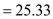Calculate the equilibrium quantity at the autarky level as follows: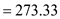Therefore, the equilibrium price and quantity are 25.33 and 273.33, respectively. Represent the demand and supply of the good, in absence of a tariff, as follows:Now, calculate the amount of the good the country will have to import as follows:a.With imposition of a tariff of 5 per unit, the domestic price increases from 10 to 15. Now, calculate the new demand and supply as follows:There is an increase in domestic production from 120 to 170, that is, production increases by 50 units. While there is reduction in demand from 350 to 325, that is, demand falls by 25 units. Calculate the welfare effect of the tariff as follows:Thus, there is a total welfare benefit of 312.5 units. b.With a production subsidy of 5, the price that the seller receives is 15 per unit instead of 10 per unit. Calculate the new supply as follows:The production rises to 170, that is, it increases by 50. But demand remains the same at 350. The consumer loss is eliminated as consumers can still consume at a price of 10. Now, Calculate the total effect from subsidy as follows:Thus, there is a gain in welfare of 375 units. c.The production subsidy produces a greater gain in welfare than the tariff because it only affects the prices in the production side and not the demand side. Consumers stay at the original consumption point and there is no distortion in the quantity demanded. However, the supply increase. This leads to an increase in the overall welfare. On the other hand, tariff affects the prices in both the production and demand side. Therefore, though the supply increases, there is a consequent fall in demand. Thus, the net gain from tariff is less than a targeted policy. d.The optimal production subsidy can be calculated by setting a goal of maximizing change in welfare of a country. Represent change in the welfare of the country as follows: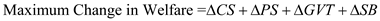Here, ? CS is the change in consumer surplus, ? PS is the change in production surplus, ? GVT is the change in government expenditure, and ? SB is the change in social benefit. Represent the new price as follows: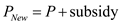Substitute P New in the supply equation as follows:Now, calculate the change in producer surplus as follows:Calculate the change in government expenditure as follows: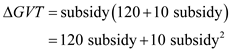Calculate the change in social benefit as follows:Substitute the value in the equation for maximum change in welfare as follows:Calculate the derivative for the above equation as follows:Therefore,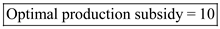.